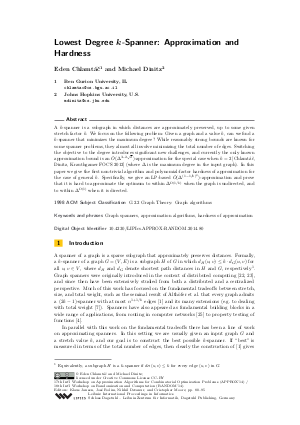Document# Lowest Degree k-Spanner: Approximation and Hardness

### Authors Eden Chlamtác, Michael Dinitz## File

LIPIcs.APPROX-RANDOM.2014.80.pdf
• Filesize: 0.51 MB
• 16 pages

## Cite As

Eden Chlamtác and Michael Dinitz. Lowest Degree k-Spanner: Approximation and Hardness. In Approximation, Randomization, and Combinatorial Optimization. Algorithms and Techniques (APPROX/RANDOM 2014). Leibniz International Proceedings in Informatics (LIPIcs), Volume 28, pp. 80-95, Schloss Dagstuhl - Leibniz-Zentrum für Informatik (2014)
https://doi.org/10.4230/LIPIcs.APPROX-RANDOM.2014.80

## Abstract

A k-spanner is a subgraph in which distances are approximately preserved, up to some given stretch factor k. We focus on the following problem: Given a graph and a value k, can we find a k-spanner that minimizes the maximum degree? While reasonably strong bounds are known for some spanner problems, they almost all involve minimizing the total number of edges. Switching the objective to the degree introduces significant new challenges, and currently the only known approximation bound is an O~(Delta^(3-2*sqrt(2)))-approximation for the special case when k = 2 [Chlamtac, Dinitz, Krauthgamer FOCS 2012] (where Delta is the maximum degree in the input graph). In this paper we give the first non-trivial algorithm and polynomial-factor hardness of approximation for the case of general k. Specifically, we give an LP-based O~(Delta^((1-1/k)^2) )-approximation and prove that it is hard to approximate the optimum to within Delta^Omega(1/k) when the graph is undirected, and to within Delta^Omega(1) when it is directed.
##### Keywords
• Graph spanners
• approximation algorithms
• hardness of approximation

## Metrics

• Access Statistics
• Total Accesses (updated on a weekly basis)
0